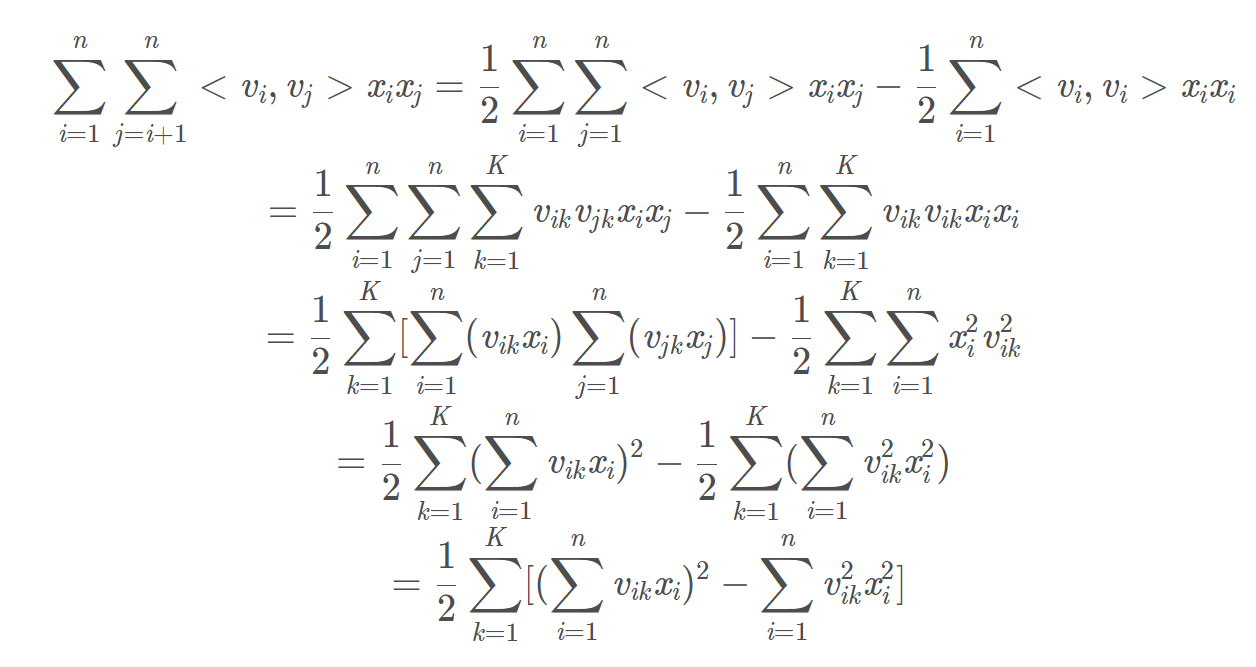### 二、特征交叉的开始——POLY2模型

$$POLY2(w,x)=w_0+\sum_{i=1}^n w_ix_i+\sum_{i=1}^{n-1}\sum_{j=i+1}^n w_{ij}x_ix_j$$

• 在处理数据时，经常采用 one-hot 编码的方法处理类别型数据，致使特征向量极度稀疏， $POLY2$ 进行无选择的特征交叉，使原本就非常稀疏的特征向量更加稀疏，导致大部分交叉特征的权重缺乏有效的数据进行训练，无法收敛。
• 权重参数的数量由 $n$ 直接上升到 $n^2$ ，极大的增加了训练复杂度。

### 三、因子分解机模型（Factorization Machine, FM）（2010年）

$$FM(w,x)=w_0+\sum_{i=1}^n w_ix_i+\sum_{i=1}^{n-1}\sum_{j=i+1}^n （\vec{v_i}\cdot \vec{v_j}）x_ix_j$$

$FM$ 通过引入特征隐向量的方式，直接把 $POLY2$ 模型 $n^2$ 级别的权重参数数量减少到了 $nk$ （$k$ 为隐向量维度， $n>>k$），极大地降低了训练开销；而且，隐向量的引入使 $FM$ 能更好地解决数据稀疏性的问题。比如在 $POLY2$ 模型中，只有当特征$x_i,x_j$ 均非零时，才能训练与之对应的 $w_{ij}$，而对于 $FM$ ，每个特征都有其对应的隐向量，减少了训练条件的苛刻性，并且对于未出现的特征组合，只要模型之前分别学习过对应的隐向量，就具备了计算其特征组合权重的能力。

$FM$ 公式二次项后继推导如下：$$\frac{∂FM}{∂ \theta}=\begin{cases}1,&\theta=w_0 \cr x_i,&\theta=w_i \cr x_i\sum_{i=1}^n(v_{ik}x_i)-v_{ik}x_i^2,&\theta=v_{ik}\end{cases}$$

### 四、特征域感知因子分解机模型（Field-aware Factorization Machines，FFM）（2015年）

$$FFM(w,x)=w_0+\sum_{i=1}^n w_ix_i+\sum_{i=1}^{n-1}\sum_{j=i+1}^n （\vec{v_{i，f_j}}\cdot \vec{v_{j,f_i}}）x_ix_j$$

#### 为什么引入Field？

 field user field(U) item field(I) clicked userId Gender_男 Gender_女 Age_[20,30] Age_[30,40] Tag_篮球 Tag_化妆品 1 1 1 0 1 0 1 0 0 1 1 0 1 0 0 1 0 2 0 1 0 1 1 0 1 2 0 1 0 1 0 1

$FFM$ 模型认为 $v_i$ 不仅跟 $x_i$ 有关系，还跟与 $x_i$ 相乘的 $x_j$ 所属的Field有关系，即 $v_i$ 成了一个二维向量 $v_{f×k}$，$k$ 是隐向量长度，$f$ 是Field的总个数。设样本一共有 $n$ 个特征, 那么 $FFM$ 的二次项有 $nf$ 个隐向量。而在 $FM$ 模型中，每一维特征的隐向量只有一个。$FM$ 可以看作 $FFM$ 的特例，是把所有特征都归属到一个 $field$ 时的 $FFM$ 模型。

### 五、FM与FFM的代码实现

#### 5.2 FFM微信支付宝